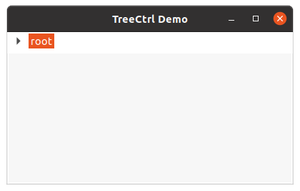Related Articles

# wxPython – GetBoundingRect() method in wx.TreeCtrl

• Last Updated : 25 Nov, 2020

In this article, we are going to learn about GetBoundingRect() method in wx.TreeCtrl. GetBoundingRect() returns the rectangle bounding the item. If textOnly is True, only the rectangle around the item’s label will be returned, otherwise, the item’s image is also taken into account. The return value may be None if the rectangle was not successfully retrieved, such as if the item is currently not visible.

GetBoundingRect() takes two arguments item and textOnly.

Attention geek! Strengthen your foundations with the Python Programming Foundation Course and learn the basics.

To begin with, your interview preparations Enhance your Data Structures concepts with the Python DS Course. And to begin with your Machine Learning Journey, join the Machine Learning - Basic Level Course

Syntax:

wx.TreeCtrl.GetBoundingRect(self, item, textOnly)

Parameters:

## Python

 `import` `wx `` ` ` ` `class` `MyTree(wx.TreeCtrl): `` ` `    ``def` `__init__(``self``, parent, ``id``, pos, size, style): ``        ``wx.TreeCtrl.__init__(``self``, parent, ``id``, pos, size, style) `` ` ` ` `class` `TreePanel(wx.Panel): `` ` `    ``def` `__init__(``self``, parent): ``        ``wx.Panel.__init__(``self``, parent) ``         ` `        ``# create tree control in window ``        ``self``.tree ``=` `MyTree(``self``, wx.ID_ANY, wx.DefaultPosition, wx.DefaultSize, ``                        ``wx.TR_HAS_BUTTONS) ``         ` `        ``# CREATE TREE ROOT ``        ``self``.root ``=` `self``.tree.AddRoot(``'root'``) ``        ``self``.tree.SetPyData(``self``.root, (``'key'``, ``'value'``)) `` ` `        ``# add item to root ``        ``item ``=` `self``.tree.AppendItem(``self``.root, ``"Item"``) ``        ``item2 ``=` `self``.tree.AppendItem(``self``.root, ``"Item"``) ``        ``item3 ``=` `self``.tree.AppendItem(item, ``"SubItem"``)``        ``item4 ``=` `self``.tree.AppendItem(item, ``"SubItem"``)``        ``item5 ``=` `self``.tree.AppendItem(item2, ``"SubItem"``)``        ``item6 ``=` `self``.tree.AppendItem(item, ``"SubItem"``)`` ` `        ``# print bound rectangle pyObject``        ``print``(``self``.tree.GetBoundingRect(item, ``False``))`` ` `        ``# expand all nodes of the tree``        ``self``.tree.ExpandAllChildren(item) ``         ` `        ``sizer ``=` `wx.BoxSizer(wx.VERTICAL) ``        ``sizer.Add(``self``.tree, ``0``, wx.EXPAND) ``        ``self``.SetSizer(sizer) `` ` ` ` `class` `MainFrame(wx.Frame): `` ` `    ``def` `__init__(``self``): ``        ``wx.Frame.__init__(``self``, parent ``=` `None``, title ``=``'TreeCtrl Demo'``) ``        ``panel ``=` `TreePanel(``self``) ``        ``self``.Show() `` ` ` ` `if` `__name__ ``=``=` `'__main__'``: ``    ``app ``=` `wx.App(redirect ``=` `False``) ``    ``frame ``=` `MainFrame() ``    ``app.MainLoop()`

Output:

`(0, 0 , 10, 10)`My Personal Notes arrow_drop_up